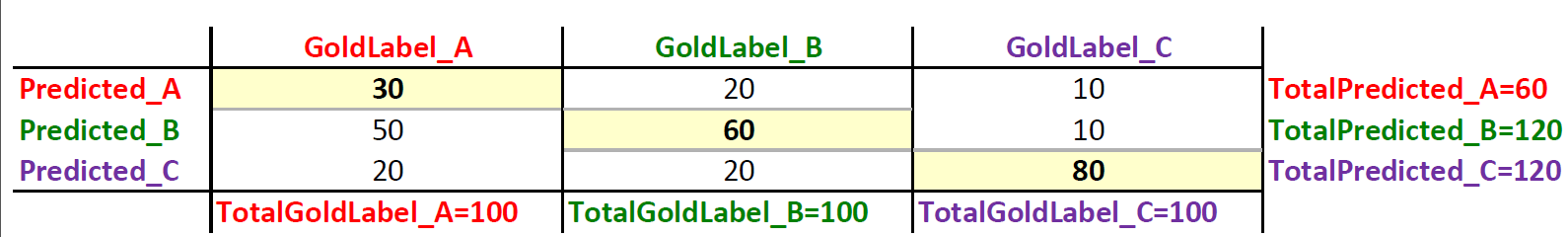## Oct 20, 2014

### Computing Precision and Recall for Multi-Class Classification Problems

In evaluating multi-class classification problems, we often think that the only way to evaluate performance is by computing the accuracy which is the proportion or
percentage of correcly predicted labels over all predictions. However, we can always compute precision and recall for each class label and analyze the individual performance on class labels or average the values to get the overall precision and recall. Accuracy alone is sometimes quite misleading as you may have a model with relatively 'high' accuracy with the model predicting the 'not so important' class labels fairly accurately (e.g. "unknown bucket") but the model may be making all sorts of mistakes on the classes that are actually critical to the application.

## What Does Precision and Recall Tell Us?

Precision:
Given all the predicted labels (for a given class X), how many instances were correctly predicted?
Recall: For all instances that should have a label X, how many of these were correctly captured?

## Computing Precision and Recall for the Multi-Class Problem

While it is fairly straightforward to compute precision and recall for a binary classification problem, it can be quite confusing as to how to compute these values for a multi-class classifcation problem. Now lets look at how to compute precision and recall for a multi-class problem.
• First, let us assume that we have a 3-class multi classification problem , with labels A, B and C.
• The first thing to do is to generate a confusion matrix as below. Many existing machine learning packages already generate the confusion matrix for you, but if you don't have that luxury, it is actually very easy to implement it yourself by keeping counters for the true positives, false positives and total number of instances for each label.This is an example confusion matrix for 3 labels: A,B and C
• Once you have the confusion matrix, you have all the values you need to compute precision and recall for each class. Note that t
he values in the diagonal would always be the true positives  (TP).

• Now, let us compute recall for Label A:

• ```= TP_A/(TP_A+FN_A)
= TP_A/(Total Gold for A)
= TP_A/TotalGoldLabel_A
= 30/100
= 0.3
```

• Now, let us compute precision for Label A:

• ```= TP_A/(TP_A+FP_A)
= TP_A/(Total predicted as A)
= TP_A/TotalPredicted_A
= 30/60
= 0.5```

• So precision=0.5 and recall=0.3 for label A. Which means that for precision, out of the times label A was predicted, 50% of the time the system was in fact correct. And for recall, it means that out of all the times label A should have been predicted only 30% of the labels were correctly predicted.

• Now, let us compute recall for Label B:

• ```= TP_B/(TP_B+FN_B)
= TP_B/(Total Gold for B)
= TP_B/TotalGoldLabel_B
= 60/100
= 0.6
```

• Now, let us compute precision for Label B:

• ```= TP_B/(TP_B+FP_B)
= TP_B/(Total predicted as B)
= TP_B/TotalPredicted_B
= 60/120
= 0.5```

• So precision=0.5 and recall=0.6 for label B. So you just have to repeat this for each label in your multi-class classification problem.

• ## The Need for a Confusion Matrix

Apart from helping with computing precision and recall, it is always important to look at the confusion matrix to analyze your results as  it also gives you very strong clues as to where your classifier is going wrong. So for example, for Label A you can see that the classifier incorrectly labelled Label B for majority of the mislabeled cases. Which means the classifier is somehow confused between label A and B. So, you can add biasing features to improve classification of label A.  In essence, the more zeroes or smaller the numbers on all cells but the diagonal, the better your classifier is doing. So tweak your features and analyze your confusion matrix !